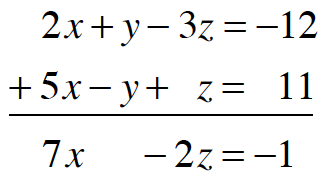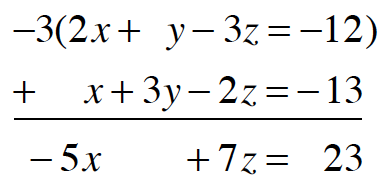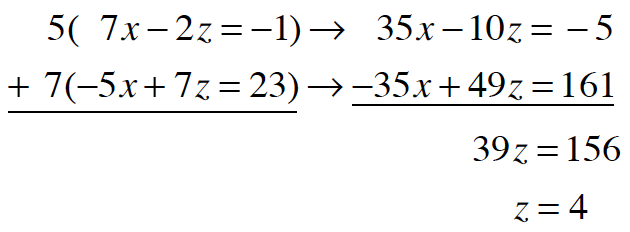### Home > CCA2 > Chapter 6 > Lesson 6.1.4 > Problem6-51

6-51.

Use the algebraic strategies you developed in today's lesson to solve the system of equations below. Be sure to check your solution. Homework Help ✎

$2x+y−3z=−12\\ 5x−y+z=11\\ x+3y−2z=−13$

Choose a pair of equations that can be added together to eliminate a variable.Choose a different pair of equations to add together to eliminate the same variable.
In this case, you will have to multiply each equation by a constant.Solve the system of equations you created in steps $1\text{ and}\ 2$ for either $x\ \text{or}\ z$.Solve for $x$ using the two-variable equation you created in either step 1 or step 2.
Then substitute the values for $x\ \text{and}\ z$ into one of the original equations to solve for $y$.

Check your solution in a different equation than the one you used to solve for $y$.